# Optimizing MeshesThis task will show you how to optimize an existing mesh, i.e. redistribute and reshape the triangles within the mesh.
This way you will obtain a more homogeneous mesh, e.g. for analysis purpose.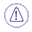• Be aware that this optimization tends to modify the shape of the model!
• The mesh must be exempt of Non Manifold problems, even on non-active areas.
You should use the Mesh Cleaner.
• Mesh Optimize is an iterative operator using edge splits and edge collapses. If an edge is too long, it is split in two.
If an edge is too short it is collapsed. Consequently, there is a minimum ratio between the minimum and
maximum edge length; otherwise an edge that has just been split could be collapsed at the next step and
then split again and so on.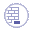Open the SmoothMesh01.CATPart from the samples directory.
You may want to display the triangles of the mesh, using the Cloud Display command.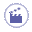1. Click Optimize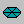and select the mesh.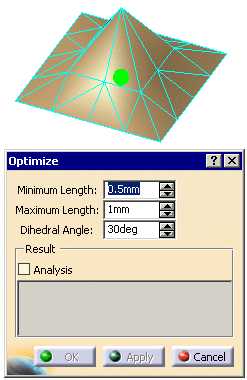2. Set the Minimum Length, Maximum Length and Dihedral Angle according to your needs.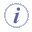Minimum Length and Maximum Length refer to the length of the edges of the triangles. The Minimum Length must be less than or equal to the half of the Maximum Length. Dihedral Angle: The dihedral angle between 2 triangles sharing an edge is the angle between their normals (note that this is different from the angle across the common edge). A greater dihedral angle indicates a sharp feature across that edge - for example if the dihedral angle is 0 it means that the 2 triangles are on the same plane. All the triangles with edges shorter than the Minimum Length will be collapsed so that their edges reach this Minimum Length. All the triangles with edges larger than the Maximum Length will be refined so that their edges reach this Maximum Length. Al the triangles with an Dihedral Angle lower than the value given will be flipped. Make sure the chosen values are coherent with your model.
3. Select the Analysis check box to display the maximum and the mean deviations
(distances between a vertex and its initial position) in the dialog box.

4. Click Apply.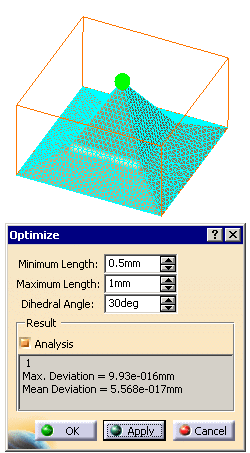5. Click OK to validate the optimization and modify the initial mesh,
or Cancel to exit the action without any modification.